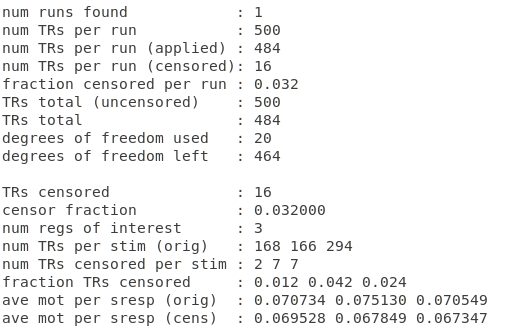# Number of censored TRs

Hi,

I need your help with understanding how to interpret the stats about censored TRs at the bottom of the output of preprocessing.
As you can see, I have 500 TRs and 3 regressors of interests. When I look at the number of TR per stims, the sum is 168+166+294=628. This is a number which is greater than 500. How is that possible?

One options is that it could have something to do with the overlap of the convolved regressors (see 1dplot attached).
In any case, how does this affect the calculation of the fraction of censored TRs per stim? (which is the info I would like to extract at the end of the day).Hi-

I believe the count of TRs per stim is from the convolved time series, which do appear to overlap a fair amount.

Note that the afni_proc.py QC (APQC) HTML does calculate the number of censored time points per stim, even providing some warning levels. Have you looked at that? There should be a QC_\${subj} directory in your AP results output, and you can open the index.html file there. There will be stats maps, alignment checks and much, much more.

Here is an older set of pages about it:
https://afni.nimh.nih.gov/pub/dist/doc/htmldoc/tutorials/apqc_html/main_toc.html

–pt

That is right. To a fair degree, afni_proc.py does not know (or attempt to figure out) the number of time points that have any stim at all in them (which themselves would overlap, since many studies have multiple stimulations within a single TR (cue, button press, etc).

In any case, the number of TRs per stim (class) refers to the number of non-zero time points in the regressor.
For descriptions, see:

``````gen_ss_review_scripts.py -help_fields
``````
• rick